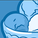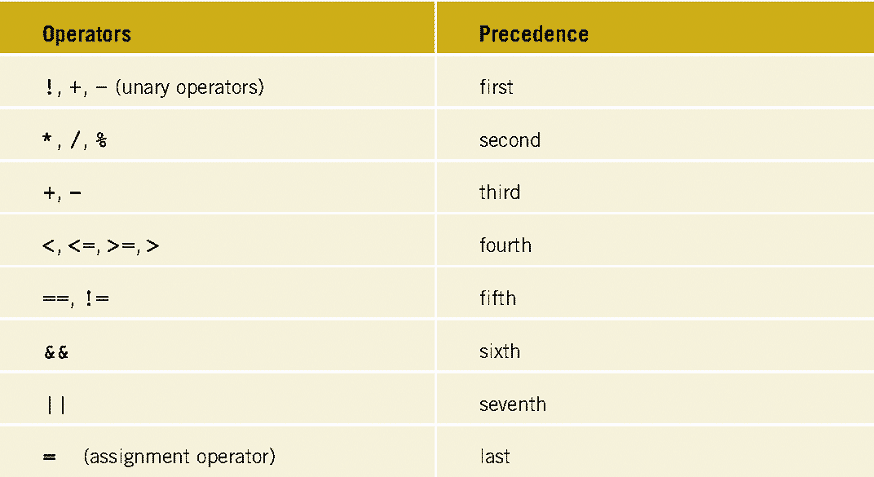# QlikView App Dev

Discussion Board for collaboration related to QlikView App Development.

cancel
Showing results for
Did you mean:Contributor III

## Calculation-Problem in diagram

Hi all,

I want to calculate a field in a diagram:

(sum(Price1)+sum(price2)) / sum(cost)

It doesn't work right: Do u have any idea how to get multiplication and division first, then addition and subtraction?

THX

1 Solution

Accepted SolutionsPartner

Do you mean this:

=Sum((Price1 + Price2)/Cost)

Although I would do this rather - it will handle nulls properly:

=Sum(RangeSum(Price1, Price2)/Cost)

Logic will get you from a to b. Imagination will take you everywhere. - A Einstein
2 RepliesMVP

What you provide the expression will work. And it works and starts from Left - Right. Ref image attacchedBefore develop something, think If placed (The Right information | To the right people | At the Right time | In the Right place | With the Right context)Partner

Do you mean this:

=Sum((Price1 + Price2)/Cost)

Although I would do this rather - it will handle nulls properly:

=Sum(RangeSum(Price1, Price2)/Cost)

Logic will get you from a to b. Imagination will take you everywhere. - A Einstein